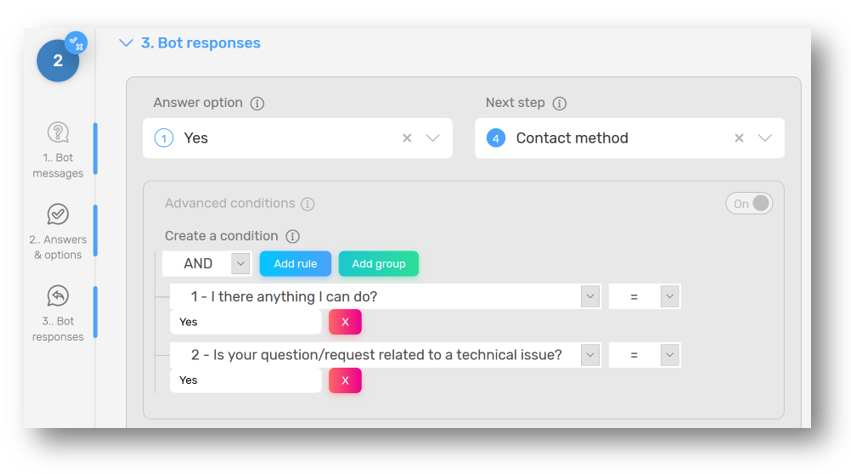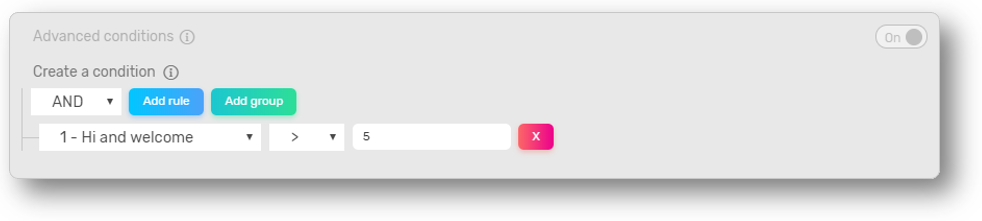For example, you can use conditioning to give your customers a promotion code if they give your feedback bot a bad review and rate your product less than 3/5. You can also add a 'Fold Out' step to show them a YouTube video they would be interested in if they indicate a specific location or a budget over 50€.

When switching on the ‘Advanced conditions’ switch in the bot response section, you are enabled to add rules. Adding conditions allows you to jump to the right step if the user’s answers are meeting definite conditions, following a special pattern or containing a specific word. That way, you can provide the right answer to behavior.## Logical conditions

Inside the different rules (or groups of rules), the different rules will be tested with these condition operators:

For questions that contain numbers or text, the logical conditions you can use are:

•  '='      → Equals to [Value].  When you want the answer to match exactly the word or definite value.Here the rule will be true only if the answer is equal to "Five"

• '!='     → Different than [Value]. When you want the answer to be different to a specific word or value.

• '>'        → Greater than [Value]. When you want the answer to be higher than a definite value.Here the rule will be true only if the answer is greater than 5, so 5 is false but 6 is not.

• >=      → Equal or higher than [Value]. When you want the answer to be equal or higher than a definite value.

• <        → Lower than [Value]. When you want the answer to be lower than a definite value.

• <=      → Equal or higher than [Value]. When you want the answer to be equal or lower than a definite value.

For text questions (when the user must write his answer), a rule can also be for an answer to contain specific words. Two options are possible:

• 'Contains (Case-sensitive)': Verify if the answer contains a specific word that also matches the upper and lower case of that word. If the word the sentence must contain is ‘Help’ and the user type ‘HELP’, the rule won’t be true.

• 'Contains (Case-insensitive)': Verify if the answer contains a specific word regardless of the upper and lower case. If the word the sentence must contain is ‘Help’ and the user type ‘HELP’, the rule will be true.

## Types of operators

Operators can either be 'AND' or 'OR'. These operators will be used when combining the rules inside a group of rules. 'AND' means that the action will be executed only if all the rules in the group are true, while 'OR' means the action will be executed if at least one of the rules in the group is true.

In the following example, If the user answers ‘Yes’ to the first question and ‘Yes’ to the second one too, then the next step will be the bot asking the user’s favourite contact method he prefers. As it is a ‘AND’ rule, if one of these two conditions is not fulfilled, then nothing will happen.## Groups (or Rules)

You are also allowed to create groups of rules to dive deeper in the conditions: If all the rules in the group A or in the group B are true, or if one group is true, a specific step is executed. Groups work just the same as the other rules, the group is just being a rule itself.

In the example below, the specific action is triggered only if one of the two groups is true (The operator is OR). For one of the groups to be true, the user must say he visits the stores more than 4 times per month and has a monthly budget equals or higher than €100 OR visit the stores more than 4 times per month and have at least 3 family members using this type of product.Need more help or looking for inspiration? Join the Quriobot community!Quriobot
Friendly & Fun
Interact & Convert